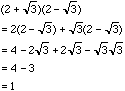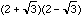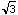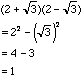Sender: "Pat" Date: Mon, 17 May 1999 11:13:39 -0700 Level: Introductory Algebra Person asking question: Student (2 + sq. root of 3) x (2 - sq. root of 3) = 1 Please show me the work. Thank you. PAT Hi Pat There are two ways to approach this problem. You can expand the left side using the distributive law or you can expand the left side by recognizing a pattern. A large part of algebra is recognizing patterns.    If you don't see the pattern then use the distributive lawThe pattern that you might have recognized is the difference of squares which says that for any numbers a and b, a2 - b2 = (a - b)(a + b) You can prove that this expression is true by expanding the right side as above. If you recognizeas the right side of the difference of squares expression with a = 2 and b =then you see immediately thatCheers, Harley Go to Math Central To return to the previous page use your browser's back button.